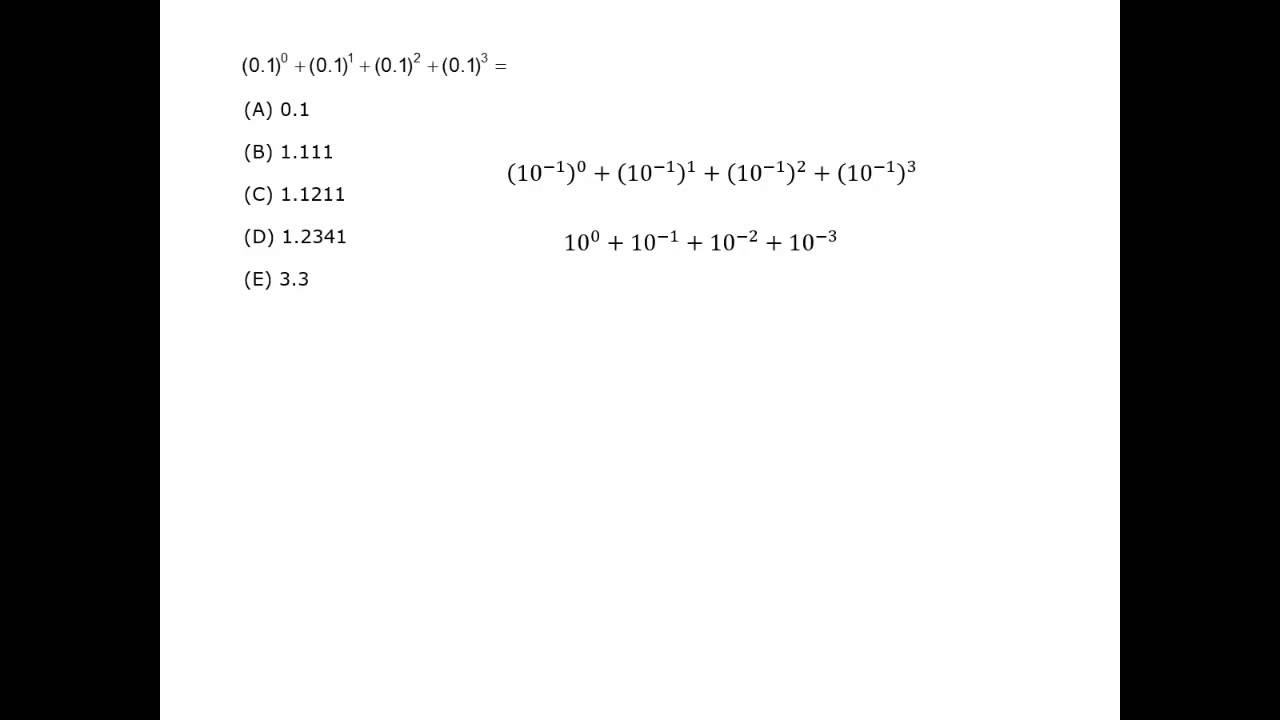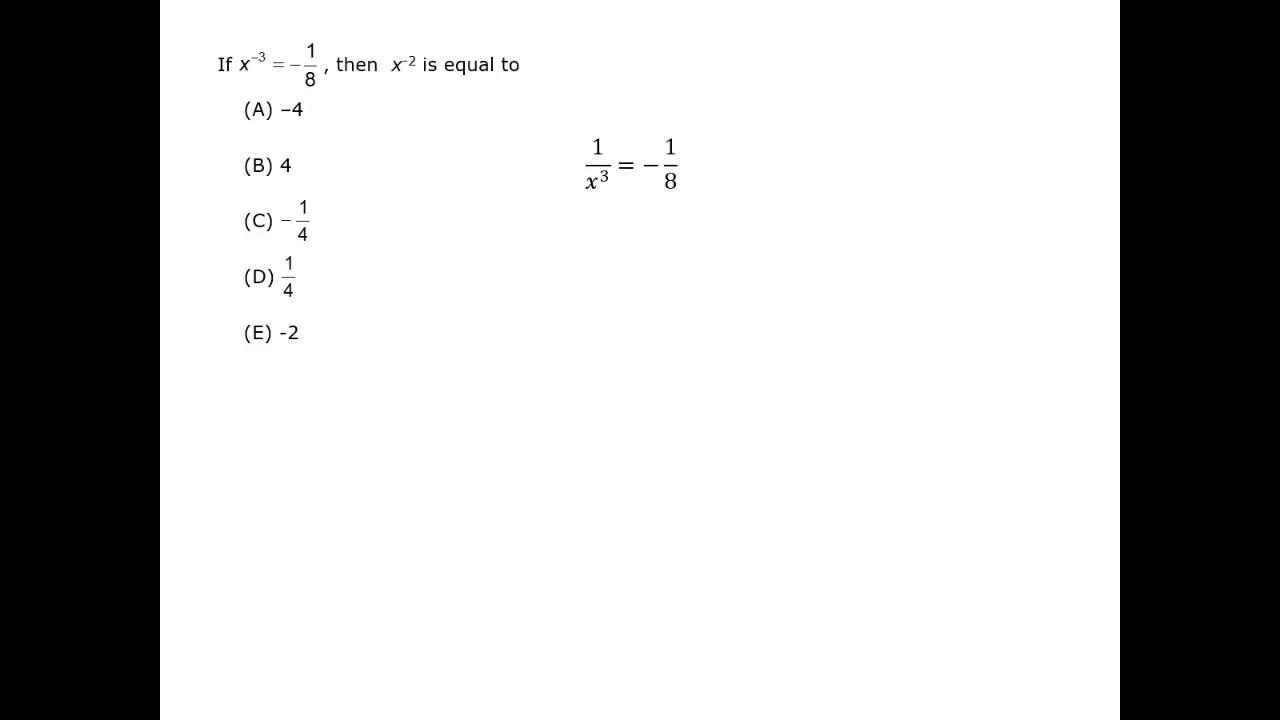## Definitions

Using an exponent is a way of expressing multiplication of a number by itself. The exponent says how many times the number, called the base, is used as a factor. An expression that has a base and exponent is called a power.

52 = 5 × 5 = 25

base = 5, exponent = 2

Numbers with the exponent 2 are called squared.  The expression 52 can be read as “five to the second power” or “five squared.”

63 = 6 × 6 × 6 = 216

base = 6, exponent = 3

Numbers with the exponent 3 are called cubed. The expression 63 can be read as “six to the third power” or “six cubed.”

34 = 3 × 3 × 3 × 3 = 81

base = 3, exponent = 4

The expression 34 is read as “three to the fourth power.”

22 = 4
32 = 9
42 = 16
52 = 25
62 = 36
72 = 49
82= 64
92 = 81
102 = 100
112 = 121
122 = 144
132 = 169
142 = 196
152 = 225

23 = 8
33 = 27
43 = 64
53 = 125
63 = 216

20 = 1
21 = 2
22 = 4
23 = 8
24 = 16
25 = 32
26 = 64
27 = 128
28 = 256
29 = 512
210 = 1,024

32 = 9
33 = 27
34 = 81
35 = 243

52 = 25
53 = 125
54 = 625

102 = 100
103 = 1,000
104 = 10,000

## Rules of Exponents

This list outlines the rules of exponents and shows an example of each.

A negative number taken to an even exponent is always a positive number.

(-2)4 = (-2) × (-2) × (-2) × (-2) = 16

(-5)-2 = \dfrac{1}{(-5)^{\displaystyle{2}}} = \dfrac{1}{((-5) × (-5))} = \dfrac{1}{25}

1) a0 = 1, a ≠ 0

50 = 1

A number to the zero power equals 1.
00 is undefined.

2) a1 = a

71 = 7

A number to the first power is equal to itself.

4) \dfrac{\textit{a}^{\displaystyle{\textit{m}}}}{\textit{a}^{\displaystyle{\textit{n}}}} = amn, a ≠ 0

\dfrac{7^{\displaystyle{5}}}{7^{\displaystyle{3}}} = 75 – 3 = 72

\dfrac{\textit{y}^{\displaystyle{6}}}{\textit{y}^{\displaystyle{4}}} = y6 – 4 = y2

Subtract exponents when dividing two terms that have the same base.

7) (\dfrac{\textit{a}}{\textit{b}})n = \dfrac{\textit{a}^{\displaystyle{\textit{n}}}}{\textit{b}^{\displaystyle{\textit{n}}}}, b ≠ 0

(\dfrac{3}{4})3 = \dfrac{3^{\displaystyle{3}}}{4^{\displaystyle{3}}}
(\dfrac{\textit{x}^{\displaystyle{5}}}{\textit{y}})2 = \dfrac{{(\textit{x}^{\displaystyle{5}})}^{{\displaystyle{2}}}}{\textit{y}^{\displaystyle{2}}} = \dfrac{\textit{x}^{\displaystyle{10}}}{\textit{y}^{\displaystyle{2}}}

The power of a quotient distributes the power to the numerator and denominator.

## Manipulating Exponents

It’s easy to manipulate exponents, but they have to have the same base.

3) am × an = am + n

32 × 33 = 32 + 3 = 35
x4 × x2 = x4 + 2 = x6

Add the exponents when multiplying two terms with the same base.

6) (ab)nanbn

(3 × 5)2 = 32 52
(x2y)3 = (x2)3y3 = x6y3

To find the power of a product, distribute the power to each factor.

5) (am)namn

(23)2 = 23 × 2 = 26
(y4)3 = y4 × 3 = y12

Multiply the exponents to raise a power to a power.

#### Common Errors

anamanm

24 – 23 is not equal to 2.

16 – 8 ≠ 2

an + ana2n

72 + 72 is not equal to 74.

49 + 49 ≠ 49 × 49

an + an + ana3n

23 + 23 + 23 is not equal to 29.

8 + 8 + 8 ≠ 512

an × amanm

22 × 23 is not equal to 26.

4 × 8 ≠ 64

an + bn ≠ (a + b)n

32 + 52 is not equal to (3 + 5)2.

9 + 25 ≠ 64

an + aman + m

24 + 23 is not equal to 27.

16 + 8 ≠ 128

A negative number taken to an odd exponent is always a negative number.

(-4)3 = (-4) × (-4) × (-4) = -64

(-2)-3 = \dfrac{1}{(-2)^{\displaystyle{3}}} = \dfrac{1}{((-2) × (-2) × (-2))}
= \dfrac{1}{(-8)} = \dfrac{-1}{(8)}

## Negative Exponents

When applying exponents to negative numbers, make sure your answer has the correct sign.

The reciprocal for a number with a positive exponent, \dfrac{1}{\textit{a}^{\displaystyle{\textit{n}}}} (n is a positive integer), is equivalent to the number with the negative exponentan.
\\[1ex]\textit{a}^{\displaystyle{-\textit{n}}} = \dfrac{1}{\textit{a}^{\displaystyle{\textit{n}}}}

Knowing these values and the rules of exponents means you can more quickly calculate other values.

For example, since 210 = 1,024, you know 212 = 1,024 × 4 = 4,096 and

2-10 = \dfrac{1}{1,024}.

8) \textit{a}^{\displaystyle{\textit{n}}} = \dfrac{1}{\textit{a}^{\displaystyle{-\textit{n}}}}

52 = \dfrac{1}{5^{-\displaystyle{2}}}

A number with a positive exponent can be written as the reciprocal for a negative exponent.

Evaluate: \dfrac{5}{3^{-\displaystyle{2}}}

### Solution

Apply the rule an = \dfrac{1}{\textit{a}^{\displaystyle{\textit{n}}}} to the denominator.

\dfrac{5}{3^{-\displaystyle{2}}} = \dfrac{5}{\dfrac{1}{3^{\displaystyle{2}}}}  …Remember that division by a fraction is multiplication by its reciprocal.

\dfrac{5}{\dfrac{1}{3^{\displaystyle{2}}}} = 5 × 32 = 45

But a leading negative sign is not affected by the exponent.

-52 = -(25) = -25

-(-4)3 = -((-4) × (-4) × (-4)) = -(-64) = 64

#### Exponents

Best viewed in landscape mode

5 questions with video explanations

100 seconds per question## Simplifying Exponential Expressions

Looking at the answers before starting to solve the problem is a good strategy for questions with exponents.  You may match the answer format by just simplifying or estimating, rather than calculating to get a value.

Simplifying may be all that is needed.  Answers will often have exponents rather than a numerical value.

An estimate needs to match the accuracy of the possible answer choices.

For any answer format, the first step is often to factor then simplify using the rules of exponents.

Remember to use Experiment as a technique if you’re not sure you remember a rule.

242 × 153 =

### Solution

Answers will often contain the exponents rather than multiplying to get a value. Find the prime factors before multiplying the terms.

242 × 153

= (2 × 2 × 2 × 3)2 × (3 × 5)3

= (23 × 3)2 × (3 × 5)3

= (23)2 × 32 × 33 × 53

= 26 × 35 × 53

Evaluate: \dfrac{8^{\displaystyle{2}}}{4^{\displaystyle{5}}}

### Solution

Find the prime factors to get the same base, then use the rule

\dfrac{\textit{a}^{\displaystyle{\textit{m}}}}{\textit{a}^{\displaystyle{\textit{n}}}} = amn

\dfrac{8^{\displaystyle{2}}}{4^{\displaystyle{5}}} = \dfrac{{(2^{\displaystyle{3}})}^{{\displaystyle{2}}}}{{(2^{\displaystyle{2}})}^{{\displaystyle{5}}}} = \dfrac{2^{\displaystyle{6}}}{2^{\displaystyle{10}}}

= 26 – 10 = 2-4

To compare to the answer choices, you can evaluate further, to get a fraction or a decimal.

2-4 = \dfrac{1}{2^{\displaystyle{4}}} = \dfrac{1}{16} = 0.0625

\dfrac{2\textit{x}\textit{y}^{\displaystyle{3}}× 9\textit{y}}{{(3\textit{x}\textit{y})}^{\displaystyle{2}}}

### Simplify:

Remember that on the GMAT, fractions might be on one line. So rewrite this as “built up”, then simplify.

Do the multiplication, then use the rule \dfrac{\textit{a}^{\displaystyle{\textit{m}}}}{\textit{a}^{\displaystyle{\textit{n}}}} = amn for each base. (This is often called cancellation.)

\dfrac{{2\textit{xy}}^{\displaystyle{3}} × 9\textit{y}}{{(3\textit{xy})}^{\displaystyle{2}}} = \dfrac{18\textit{x}{\textit{y}^{\displaystyle{4}}}}{9{\textit{x}^{\displaystyle{2}}{\textit{y}^{\displaystyle{2}}}}} = \dfrac{2{\textit{y}^{\displaystyle{2}}}}{\textit{x}}

Factor.

72 + 75

### Solution

Factor by “undoing” using the distributive property.

72 + 75 = 72(1 + 73)

Factor.

32 + 122

### Solution

Factor by “undoing” using the distributive property.

32 + 122 = 32 + (3 × 4)2

= 32 + 32 42 = 32(1 + 42)

If x = 52 + 53 + 54 + 55, what is the greatest prime factor of x?

### Solution

Factor to find all the prime factors.

52 + 53 + 54 + 55

= 52(1 + 5 + 52 + 53)

= 52 (1 + 5 + 25 + 125)

= 52 (156)

= 52 (2 × 2 × 3 × 13)

So the greatest prime factor is 13.

A microorganism has a length of 5-7 meters. If 625 microorganisms of this length are arranged in a line, what is the length of the line in millimeters? (1m = 100 cm, 1 cm = 10 mm)

### Solution

Factor 625.

625 = 252 = (52)2 = 54

Multiply.  Use the rule
am × an = am + n

(5-7)(54) = 5-3 = \dfrac{1}{5^{\displaystyle{3}}} = \dfrac{1^{\displaystyle{3}}}{5^{\displaystyle{3}}}

= (\dfrac{1}{5}) × (\dfrac{1}{5}) × (\dfrac{1}{5})

= 0.2 × 0.2 × 0.2 = 0.008

Convert from meters to millimeters. 1 m = 100 cm, 1 cm = 10 mm, therefore
1 m = 1,000 mm (or 1 mm = 10-3 m), so multiply both sides by 1,000.

0.008 m = 8 mm

## Powers

Note: Powers are covered more completely in Section 6, Exponents.

Writing a number raised to a power is a way of expressing multiplication of a number by itself. A power has two parts, the exponent and the base. The exponent says the number of times the base is used as a factor.

64

### Solution

64 = 6 × 6 × 6 × 6 = 1,296

base = 6, exponent = 4

52

### Solution

52 = 5 × 5 = 25

base = 5, exponent = 2

43

### Solution

43 = 4 × 4 × 4 = 64

base = 4, exponent = 3

The expression 64 is read as “six to the fourth.”
Two powers have special names.  Powers with the exponent 2 are called “squared,” since it can imply area.  The expression 52 can be read as “five to the second power” or “five squared.”
Powers with the exponent 3 are called “cubed,” since it can imply volume.  The expression 43 can be read as “four to the third power” or “four cubed.”

### Powers of negative numbers

When using exponents with negative numbers, make sure your answer has the right sign. Pay careful attention to whether a negative sign is inside or outside of parentheses.
(-4)3 = (-4) × (-4) × (-4) = -64
(-5)2 = (-5) × (-5) = 25
but     -52 = -(5 × 5) = -25

#### Exponents

Best viewed in landscape mode

5 questions with video explanations

100 seconds per question